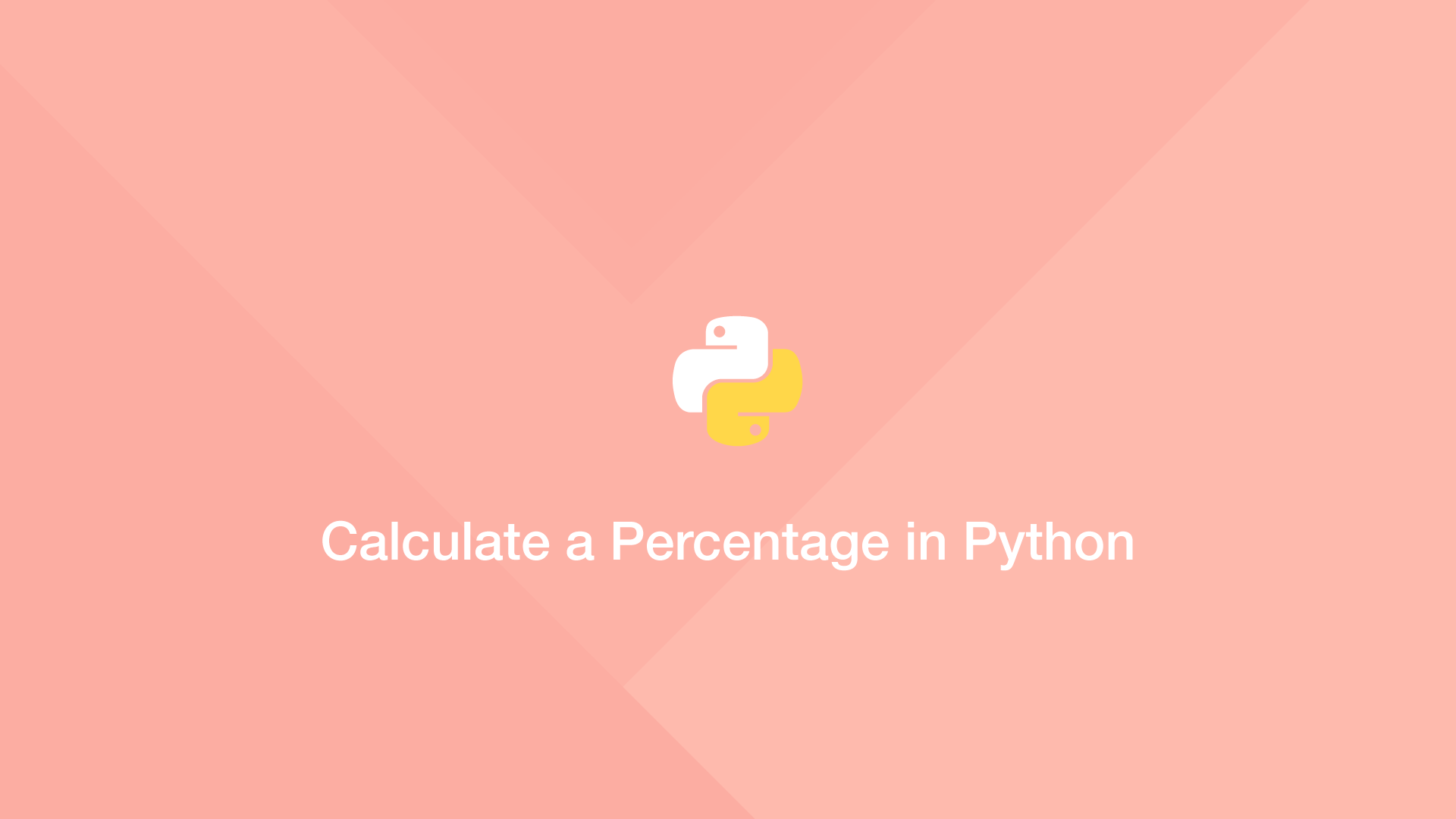# How to Calculate a Percentage in PythonTo get a percentage of two numbers in Python, divide them using the division operator (`/`) then multiply the result by 100 using the Python multiplication operator (`*`).

To demonstrate this, let's get the percentage of 3 out of 9 using Python.

``````percent = 3 / 9 * 100

print(percent)
``````
``````33.33333333333333
``````

## Python Percentage Function

If you need to get percentages many times in your program, it may be worth creating a small helper function.

``````def getPercent(first, second, integer = False):
percent = first / second * 100

if integer:
return int(percent)
return percent

print(getPercent(3, 9))
``````
``````33.33333333333333
``````

With the above function, to get an integer back pass `True` as the third argument.

``````def getPercent(first, second, integer = False):
percent = first / second * 100

if integer:
return int(percent)
return percent

print(getPercent(3, 9, True))
``````
``````33
``````

#### Related Tutorials### How to Calculate Mean, Median, Mode and Range in Python

October 07, 2020### How to Calculate Exponent in Python

May 28, 2021### How to Calculate a Square Root in Python

May 12, 2021### Calculate Euclidean Distance in Python

October 01, 2021### How to Tune PHP-FPM to Improve Performance on Server

October 22, 2021June 29, 2021